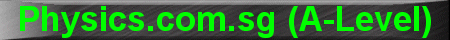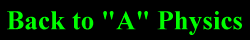(New tips are continually added to these pages.  Check back in a few months' time for more)

TOPIC 10:    Electric Fields, Electrostatics and Capacitors

Tip 1:

One capacitance equation that is very important but occasionally left out of lecture notes, is the capacitance of a parallel plate capacitor:

C = eA/d

where C is the capacitance, e the permittivity of the dielectric between the plates, A the area and d the separation between plates.

This equation is especially useful when they ask you what happens to the potential difference across a charged parallel-plate capacitor, after being disconnected from the battery,  if the separation between the plates is increased.

With this equation, we know that if d increases, C will decrease.  Since an isolated capacitor has constant charge Q, the potential difference will increase (since V = Q/C).

Without this equation, you will have to reason that since the pair of plates contain opposite charges (+ and -), they attract each other so in order to pull them further apart, you will have to do work against this attractive force.  The work done by you is converted into an increase in the stored potential energy in the capacitor, and since electrical potential energy stored in a capacitor U = ˝QV, and Q is constant in an isolated capacitor, hence V should also increase.

Under intense examination conditions, its usually easier to just plug in the equation.